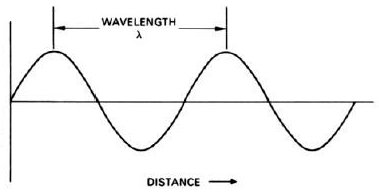A# wavelengthWavelength is the distance between successive peaks (or troughs) of a wave. Wavelength is related to the frequency and speed of a wave by the equation:

speed = wavelength × frequency.

For example, a radio wave of wavelength 300 meters traveling at 300 million meters per second (the speed of light) has a frequency of 1 MHz. The wavelength of a visible light wave, which can range from 390 to 780nm (nanometers), determines its color.